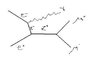# Not back-to-back emission of decay products

• malawi_glenn
In summary, the data shows that muons are not emitted back to back in the LHC. There is a small deviation from this behavior.f

#### malawi_glenn

Homework Helper
Gold Member
2022 Award
Hi!

I am reading about the $Z^0$ production and decay processes at the moment.

I have understand that the $Z^0$ is produced at rest, and when it decays to two leptons (or quarks) they must be emitted back to back to conserve momentum.

But when I look at real and simulated data, the muons are not emitted back to back, there is a small deviation.

I just wonder "WHY"? Have I missed something fundamental?

I mean, I don't think it has to do with the bending in the central detector magnetic field.

http://www.particle.kth.se/zlab/project/images/event11/event11_side.gif [Broken]

Last edited by a moderator:
Neither Z is produced at rest. Why do you think they are?

Neither Z is produced at rest. Why do you think they are?

Ok so the explanation is that the Z is not produced in rest?

I can't see it =(

using four-vectors:

$$p(e^+) + p(e^-) = E_{cm} + \vec{0} = 2E_{beam} = p_Z$$

So the Z boson's 3-momenta is equal to 0. I assue that the beams are totally head-on (same energy)

"I have understand thatif/in the frame in which the Z0 is produced at rest, then/there when it decays to two leptons (or quarks) they must be emitted back to back to conserve momentum."

I don't know what kind of event your first link is. But the 2nd one is LHC in the lab frame. The cms frame of the relevant partons that make up your process (i.e. ignoring the remants of the initial protons) does not have to coincide with the lab frame.

EDIT: Since I am late with my response: Ebeam is the energy of the proton beam (again@LHC) but you effectively collide quarks and gluons; not the whole protons.

Ok, then I see why they are not back to back @ LHC :)

But the first image is electron-postiron @ LEP.

In lab-frame if one has equal energy beams, then Z must be produced at rest also in lab-frame? That is what my equation above refers to, sorry if I wasn't clear enough.

Note that in the 1st link in addition to what supposedly is the two lepton tracks there is a green quad on the right side that indicates a detector signal and that the header sais "Hcal(N=2, SumE=4.5)" which might mean hadron calorimeter. Spontaneously from that I'd suspect that the process of the event is not "e+ e- -> s-channel Z -> mu+ mu-" but something different - but that's only a guess of mine.

EDIT: I've taken a look at the standard LEP91 setup of the Sherpa event generator. That setup includes processes with up to two QCD vertices. Assuming it wasn't put there just for fun that strengthens my idea that the missing p comes from hadronic stuff.

Last edited:
In lab-frame if one has equal energy beams, then Z must be produced at rest also in lab-frame?

No. That would be true only if the beam energy were half the Z mass. As you can see from the e+e- event display, the beam energy is 65.129 GeV, significantly above half the Z mass.

No. That would be true only if the beam energy were half the Z mass. As you can see from the e+e- event display, the beam energy is 65.129 GeV, significantly above half the Z mass.

No. That would be true only if the beam energy were half the Z mass. As you can see from the e+e- event display, the beam energy is 65.129 GeV, significantly above half the Z mass.

Timo: Ok, but if the beam have 65.129 GeV, the Z boson will be emited with some kinetic energy. But what will be emitted to compensate for the momentum violation? (since we must have overall momentum conservation here?)

V-50: So what reaction can give away two muons and a hadronic signal in an ee-bar collider @ 65GeV beam energy? :S

What reaction can give away two muons and a hadronic signal in an ee-bar collider @ 65GeV beam energy? :S

e+e- goes to Z + something else. The most likely "something else" is a photon going down the beampipe. In fact, you can see it in the picture - it's the green thing on the right. It says 32.8 GeV of energy was detected in the forward calorimeter: 65*2 - 33 = 97 GeV, pretty close to 91 GeV.

e+e- goes to Z + something else. The most likely "something else" is a photon going down the beampipe. In fact, you can see it in the picture - it's the green thing on the right. It says 32.8 GeV of energy was detected in the forward calorimeter: 65*2 - 33 = 97 GeV, pretty close to 91 GeV.

Well yes that is of course possble, so the remaining 6GeV could be kinetic energy of the Z.

So the feynman - diag would be: https://www.physicsforums.com/attachment.php?attachmentid=13824&stc=1&d=1209838584

?

#### Attachments

•zdiag.jpg
5.3 KB · Views: 585
Yes, a diagram like that.

Yes, a diagram like that.

cool!Thanx a lot Van-50 and Timo! See you around, and enjoy PF and life